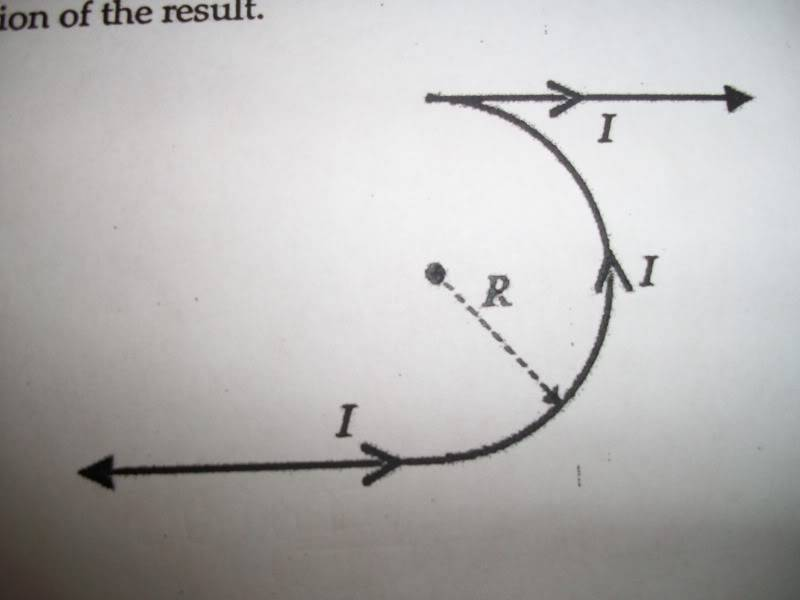# Magnetic fied at center of semicircle Ampere's Law Biot-Savart Law

sweetdion

## Homework Statement

A long wire is bent into semicircle of radius R at its center and continues on to infinity in both direcions with the straight segments remaining parallel, as shown in the figure below. Use your knowledge of superposition, Ampere's Law, and the Biot-Savart Law to determine the magnetic field at the center of the semicircle by way of the following steps:

a) determine the contribution due to the semicicular wire segment
b) determine the conribution of each straight wire segment by considering them to be semi-infinite using symmetry, as they each represent "half" of an infinite wire.
c) determine the vector sum of these three contributions, including both magnitde and direction of the result

dB=uI/2(pi)R
B = μ0I/2(pi)R

## The Attempt at a Solution

a) The magnetic field at the point P due to the wire of radius 'R' is
B = (1/2)(μ0I / 2R)
b) For an infinite wire, B = μ0I/2(pi)R
c) Sum gives: μ0piI+2μ0I/4(pi)R

correct?

sweetdion
I just need to know if this right?

Staff Emeritus
Homework Helper
You left out the figure. It is difficult to see how the straight segments are oriented without a figure. Are the two straight segments collinear or just parallel? Are they at right angles to the plane of the semicircle, or do they lie within the plane of the semicircle? Or are they oriented some other way?

Remember that B is a vector, so you need to follow the rules of vector addition. You can't just add up the expressions for each wire section, unless B happens to point in the same direction for each section.

Last edited:
sweetdion
apologies.--sweetdion

Staff Emeritus
Homework Helper
Okay, that helps. Thanks!

## The Attempt at a Solution

a) The magnetic field at the point P due to the wire of radius 'R' is
B = (1/2)(μ0I / 2R)
b) For an infinite wire, B = μ0I/2(pi)R
c) Sum gives: μ0piI+2μ0I/4(pi)R

correct?
Sorry, that's not correct. (Though you are correct about B for the semicircle, and an infinite wire.)

Here are some questions for you, to help you reason things through:

1. What is B for each half-infinite wire?

2. What is the direction of B for each section of wire (the lower-left half-infinite piece, the semicircle, and the upper-right half-infinite piece)?

3. How do the three B's you have add up, realizing that they are vectors and might point in different directions?

Hope that helps.

sweetdion
Okay, that helps. Thanks!

Sorry, that's not correct. (Though you are correct about B for the semicircle, and an infinite wire.)

Here are some questions for you, to help you reason things through:

1. What is B for each half-infinite wire?
I just divide the original equation by 2 to get B=u0I/4PiR

2. What is the direction of B for each section of wire (the lower-left half-infinite piece, the semicircle, and the upper-right half-infinite piece)?
Right hand rule gives
Lower Left: Up
Semicirle: Left
Upper Right: Up

3. How do the three B's you have add up, realizing that they are vectors and might point in different directions?

I need to know if I'm right so far to do this part.

Homework Helper
I think you need to check #2. The semicircle doesn't induce a leftward-pointing field, nor does the upper right segment induce an upward field.

sweetdion
Let me try agian,

Lower Left: Up
Semicirle: Right
Upper Right: Down

I don't think they can be coming into or out of the paper because it seems way to simple for that.

Staff Emeritus
Homework Helper
Lower Left: Up
Semicirle: Right
Upper Right: Down

I don't think they can be coming into or out of the paper because it seems way to simple for that.
You're correct for the lower-left and upper-right parts, but not for the semicircle.
Hint for the semicircle: imagine a very short wire section within the arc. What is the direction of B due to that short section?

sweetdion
okay so out of the page

sweetdion
The up and down components of the total B cancel giving that the Btot=uI/2PiR out of the page

Staff Emeritus